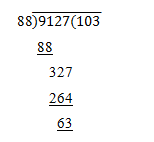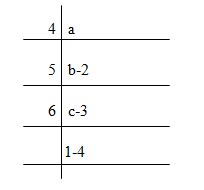# Number Systems ( 11 to 15 )

#### Prev     Next

Number Systems ( 11 to 15 ):

# APTITUDE QUESTIONS (NUMBER SYSTEMS):

11. Which natural number is nearest to 9127 which is completely divisible by 88?

A)    9152

B)    9240

C)    9064

D)    9184

E)    None of these

Explanation:

After dividing we get,∴ required number = 9127 + (88-63) = 9127 + 25 = 9152

12. How many 3-digit numbers are completely divisible by 5?

A)    149

B)    180

C)    151

D)    166

E)    None of these

Explanation:

3-digit numbers divisible by 6 are:

100, 105, 115,…., 995

This is an A.P. in which a = 100, d = 5 and l = 995

Let the number of terms be n. Then, tn = 995

∴ a +(n – 1)d = 995 => 100 + (n – 1) * 5 = 995

• 5 * (n – 1) = 895 => (n – 1) = 179 => n = 180

∴ Number of terms = 180

13. The sum of all two digit numbers divisible by 7 is

A)    1135

B)    1245

C)    1030

D)    945

E)    None of these

Explanation:

Required numbers are 14, 21, 28, …., 98.

This is an A.P. in which a = 14, d = 7 and l = 98.

So, let the number of terms in it be n. Then,

tn = 98 => a + (n – 1)d = 98

• 14 + (n – 1) * 7 = 98 => (n – 1) * 7 = 84 => (n – 1) = 14 => n = 15
• Required sum = (n/2)(a + l) = 15/2(12+96)=(9*105) = 945

14. Which one of the following is the common factor of (4743 + 4343) and (4747 + 4347)?

A)    (47 – 43)

B)    (47 + 43)

C)    (4743 + 4343)

D)    (4747 + 4347)

E)    None of these

Explanation:

If ‘n’ is odd, (xn + an) then it is always divisible by (x + a)

∴ each one of (4743 + 4343) and (4747 + 4347) is divisible by (47 + 43).

15. A number was divided successively in order by 4, 5, and 6. The remainders were respectively 2, 3 and 4. The number is:

A)    214

B)    476

C)    954

D)    1908

E)    None of these

Explanation:∴ c = 6 * 1 + 4 = 10

b = 5 * c + 3 = 5 * 10 + 3 = 53

a = 4 * b + 2 = 4 * 53 + 2 = 214